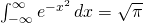## Integral of the Week #2

July 22nd, 2008 | Categories: general math, Problem of the week | Tags:

The second Integral Of The Week (IOTW) is rather different from the first in that I am going to give you the evaluation. Your task is to prove it.But WAIT! Almost every time I have seen this integral evaluated, it has been done by squaring it and converting to polar co-ordinates and that’s the one method of evaluation you can’t use for this particular challenge. I am looking for more ‘interesting’ proofs. Have fun.

Solutions can be posted in the comments section or sent to me by email (obtaining my email address is another puzzle for you to solve) and will be discussed in a future post. Feel free to send your solution in just about any format you like – plain text, uncompiled Latex, PDF, postscript, Mathematica, ODF, even Microsoft Word. When I get around to posting the solutions I will attempt to standardize them (to PDF probably).

Update: Several solutions have been posted in the comments.

1. We construct the normal distribution as the limit of the binomial probability distributions. This will automatically prove the normal distribution is a probability distribution, which will give us the desired integral.

Consider the binomial distribution B(n, 0.5)(x). Its standard deviation is 0.5SQRT(n), so we’re concerned with the function B(n, 0.5)(0.5SQRT(n)x + 0.5n)). At an integer x, the value of B(n, 0.5) is n!/(x!(n-x)!2^n), so for x + SQRT(n) an integer multiple of 2SQRT(1/n), the probability density we need is p(n, x) = SQRT(n)n!/(y!(n-y)!2^(n+1)) where y = (x + SQRT(n))SQRT(n)/2 = n/2 + xSQRT(n)/2.

Consider the value of (n-k)!(n+k)!/n!n!. It’s equal to (n+k)/n * (n+k-1)/(n-1) * … * (n+1)/(n-k+1) = (1 + k/n)(1 + k/(n-1))…(1 + k/(n-k+1)). Let k ~ mSQRT(n), m much smaller than SQRT(n). For large n, mSQRT(n)/(n – SQRT(n)) = m/(SQRT(n) – 1) = m/SQRT(n) + m/(n – SQRT(n)) and 1/n goes to 0 in all monomials. So the value of the expression approaches (1 + m/SQRT(n))^mSQRT(n) which approaches e^(m^2). In our case, y = n/2 + xSQRT(n/2)/SQRT(2), turning the function into e^(-x^2/2)*SQRT(n)n!/(n/2)!(n/2)!2^(n+1).

It suffices to find the constant, i.e. the limit of SQRT(n)n!/(n/2)!(n/2)!2^(n+1) as n goes to infinity. Replacing n by n+2 multiplies the expression by SQRT((n+2)/n)(n+1)(n+2)/(n+2)^2 = SQRT((n+2)/n)(n+1)/(n+2). Squaring, we get ((n+2)/n)(n+1)^2/(n+2)^2 = (n+1)^2/n(n+2). It suffices to prove that the limit of the product of (n+1)^2/n(n+2) over all even n is 4/pi (i.e. that the limit of Prod(n(n+2)/(n+1)^2) is pi/4). That will make the square of the limit we need to find equal to 4/8pi = 1/2pi as desired…

2. Thanks for the solutions guys – I haven’t had chance to work through them in detail yet but I am sure you haven’t made any mistakes ;)

I wonder what other approaches people might come up with?

3. Another way of doing this is taking the integral of e^(-x^2), and letting u = x^2 with du = 2xdx = 2SQRT(u)dx. This turns the integral into the integral of 0.5u^(-0.5)e^(-u). But we know that Gamma(0.5) = SQRT(pi) using the beta function, so we are done.

4. Hi Alon,

The problem with turning the integral into Γ(1/2) is that you then need to evaluate Γ(1/2), which is normally done by turning it back into a Gaussian integral again!
But, as you hinted, Γ(1/2) can be obtained from the Beta function identity
B(x,1−x) = Γ(x)Γ(1−x) = π csc(πx) evaluated at x=1/2. This identity is proved via contour integration (and a version is available on my wiki, via my homepage).

5. Hi Simon,

In a book I have (Angus E. Taylor, Advanced Calculus, Blaisdell Publishing Company, 1955) there are a series of two exercises to prove the reflection formula Γ(x)Γ(1−x) = π csc(πx) within the reals.

Exercise 10 (page 683). Show (…) that B(a,1-a)=int_{0}^{1} (x^(a-1)+x^(-a))/(1+x) dx.

Exercise 11 (page 683). Use the geometric series for (1+x)^(-1) in Exercise 10 and integrate term by term. (…) Show that B(a,1-a)= π csc(πa).

6. I’ve just posted (in Portuguese) the proof of the refection formula that I mentioned in my last comment on August 20, 2008.